# Practical Geometry Class 7 Notes: Chapter 10

According to the CBSE Syllabus 2023-24, this chapter has been removed from NCERT Class 7 Maths textbook.

## Lines That Don’t Meet

### Method of construction of a line parallel to a given line, using only a sheet of paper

1. Take a piece of paper.
2. Fold it in half and unfold the line l. Mark a point A on paper outside l.
3. Fold the paper perpendicular to the line such that this perpendicular passes through A. Name the perpendicular AN.
4. Make a fold perpendicular to AN through point A. Name the new perpendicular line as m.
5. Now,  l || m.

### Steps of construction of a line parallel to a given line

1. Take a line l and a point A outside l.
2. Take any point B on l and join it to A.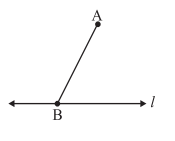3. With B as the centre and a convenient radius, cut an arc on l at C and BA at D.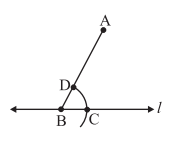4. With A as the centre and same radius as in Step 3, cut an arc EF to cut AB at G.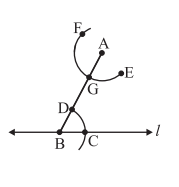5. Measure the arc length CD by placing pointed tip of the compass at C and pencil tip opening at D.
6. With this opening, keep G as centre and draw an arc to cut arc EF at H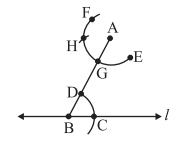7. Join AH to draw a line m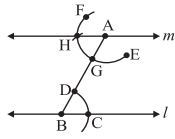∠ABC and ∠BAH are alternate interior angles. Therefore, m || l

To know more about Construction of a Parallel Line, visit here.

## Let’s Build Triangles

### Classification of triangles based on sides and angles

Triangles can be classified based on their:

1. SIDES:
• Equilateral triangle: All three sides are equal in measure.
• Isosceles triangle: Two sides have equal measure.
• Scalene triangle: All three sides have different measures.
2. ANGLES:
• Acute triangle: All angles measure less than 900.
• Obtuse triangle: One angle is greater than 900.
• Right triangle: One angle is 900.

#### For more information on Classification of triangles based on Sides and Angles, watch the below video.To know more about Classification of Triangles, visit here.

### Important properties of triangles

1. The exterior angle is equal to the sum of interior opposite angles.
2. The sum of all interior angles is 180°
3. Sum of the lengths of any two sides is greater than the length of the third side.
4. Pythagoras theorem: In any right-angled triangle, the square of the hypotenuse is equal to the sum of the squares of the other two sides.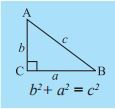To know more about Properties of Triangles, visit here.

Triangles can be constructed if any of the following measurements are given

1. Three sides.
2. Two sides and an angle between them.
3. Two angles and a side between them.
4. The hypotenuse and a leg in case of a right-angled triangle.

Construction of triangles given a criterion are listed below:

• ### Construction of a triangle with SSS criterion.

• Construct a triangle ABC, given that AB = 4.5 cm, BC = 5 cm and AC = 6 cm.
Steps:
1. Make a rough sketch for your reference
2. Draw a line segment BC = 5 cm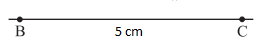3. With B as centre, draw an arc of radius 4.5 cm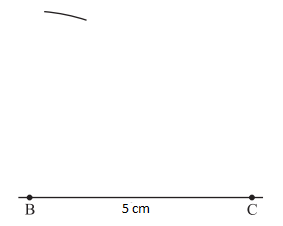4. With C as centre, draw an arc of radius 6 cm and cut the previous arc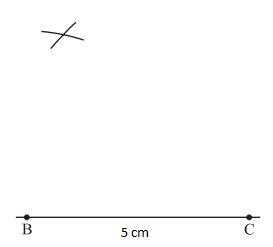5. Mark the point of intersection of arcs as A. Join AB and AC. ΔABC is now ready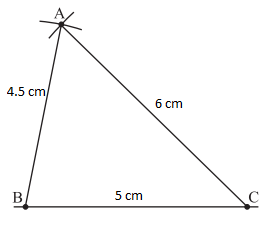• Note: SSS congruency rule:-  If three sides of one triangle are equal to the corresponding three sides of another triangle, then the two triangles are congruent
• ### Construction of a triangle with SAS criterion

• Construct ΔPQR with QR = 7.5 cm, PQ = 5 cm and ∠Q = 600.
Steps:
1. Make a rough sketch for your reference
2. Draw a line segment QR = 7.5 cm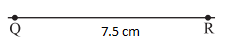3. At Q, draw QX making 600 with QR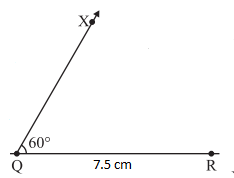4. With Q as centre, draw an arc of radius 5 cm. It cuts QX at P.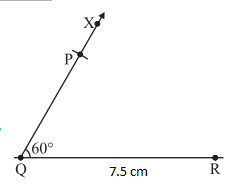5. Join AB. ΔPQR is now ready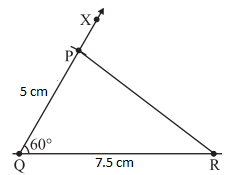• ### Construction of a triangle with ASA criterion

• Construct ΔXYZ with ∠X = 300, ∠Y = 1000 and XY = 5.8 cm.
Steps:
1. Make a rough sketch for your reference
2. Draw XY = 5.8cm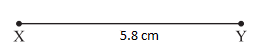3. At X, draw a ray XP making an angle of 300 with AB.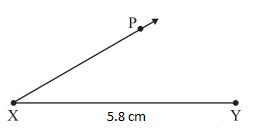4. At Y, draw a ray YQ making an angle of 1000 with XY.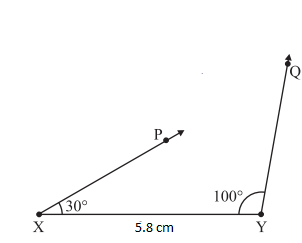5. The point of intersection of the two rays is Z.
6. ΔXYZ is now completed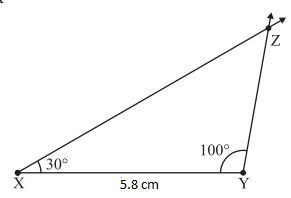• ### Construction of a triangle with RHS criterion

• Construct ΔLMN, where ∠M = 900, MN = 8cm and LN = 10 cm.
Steps:
1. Make a rough sketch for your reference
2. Draw MN = 8 cm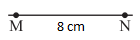3. At M, draw MX ⊥ MN.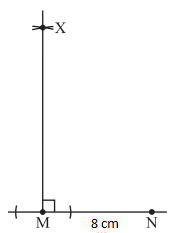4. With N as centre, draw an arc of radius 10 cm to cut MX at L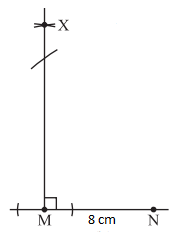5. Join LN.
6. ΔLMN is now completed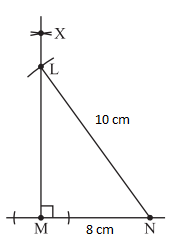To know more about Construction of triangles, visit here.

## Basics of Practical Geometry

#### For more information on Basics of Practical Geometry, watch the below video.### Introduction to Constructions of basic figures

Basic constructions:

– To draw a line segment of given length
– a line perpendicular to a given line segment
– an angle
– an angle bisector
– a circle

• In geometry, you have already studied basic constructions.
• To recap, some of them are:
• Drawing a line segment of given length
• Drawing a line perpendicular to a given line segment.
• Angles
• Angle bisectors
• Circles
• Tools used for simple constructions are ruler, protractor and a compass.

## Frequently Asked Questions on CBSE Class 7 Maths Notes Chapter 10 Practical Geometry

Q1

### What is the meaning of congruence?

The quality or state of agreeing, coinciding, or being congruent.

Q2

### What is an angle bisector?

Angle bisector is the line or line segment that divides the angle into two equal parts.

Q3

### What is a scalene triangle?

If none of the three sides of a triangle are equal to each other, then it is called a Scalene triangle.